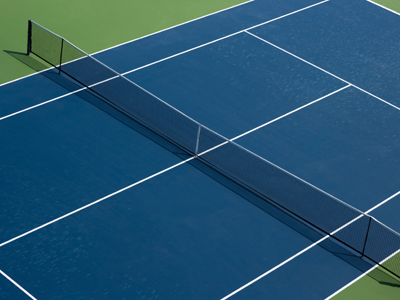The length of the perimeter of a rectangle (such as the above tennis court) = twice the length + twice the width.

# Perimeter and Area (Difficult)

Perimeter and area is all about measuring the size of shapes. This is the last section of our 11-plus Maths quizzes on perimeter and area so the questions are that little bit more difficult.

In your Maths lessons, you will be concentrating on working with flat shapes to find perimeters and areas. Objects in real-life also have perimeters and areas too. Think of a cricket pitch. It has a specific length and width - and therefore you would be able to work out both perimeter and area.

On your travels this week, see how many objects you can spot where the perimeter could be found quite easily. For some objects, you'd only need a ruler.

These are the hardest of our quizzes on perimeter and area. If you play all three sections and get all the questions right, you're an expert!

1.
Which one of the given statements is correct?
The shortest side of a right angled triangle is called the hypotenuse
The perimeter of a circle is called the circumference
π = 237
The area of a rectangle = length + width
The hypotenuse is the longest side
2.
Which one of the given statements is correct?
There are 100,000 mm2 in 1 m2
There are 1,000,000 cm2 in 1 m2
The area of a triangle = 12 × base × height
To change from square centimetres to square millimetres, you have to divide the square centimetres by 100
If you didn't get this correct, write it down and remember it!
3.
Which one of the given statements is correct?
The length of the perimeter of a polygon is found by multiplying the length of one of its sides by the number of sides
The perimeter of a circle is called the circumscribe
See the previous quizzes on perimeter and area
4.
Which one of the given statements is correct?
The length of the perimeter of a rectangle = twice the length + twice the width
A rectangle has three edges
The area of a rectangle = 12 × base × height
The fewer sides that a shape has the smaller its area
A triangle has three edges
5.
Which one of the given statements is correct?
There are 1,000 mm2 in 1 m
A hexagon has five sides
A 2-D shape doesn't have a volume
If you increase the perimeter of a 2-D shape, the area decreases
A hexagon has six sides
6.
Which one of the given statements is correct?
The area of a triangle = base × height
A cuboid has twelve faces
A heptagon has six sides
If you double the radius of a circle, the circumference doubles
See the previous quizzes on perimeter and area
7.
Which one of the given statements is correct?
A cuboid has six faces
A cube has 14 edges
The circle is the only 2D shape that has a curved perimeter
A quadrilateral has at least five sides
A quadrilateral has only four sides
8.
Which one of the given statements is correct?
The length of the perimeter of a regular polygon is found by multiplying the lengths of its sides with each other
The length of the perimeter of a regular polygon is found by multiplying the length of one of its sides by the number of sides
A 2-D shape has a volume
The length of the perimeter of a rectangle = length + width
A 3-D shape has volume
9.
Which one of the given statements is correct?
To change from square millimetres to square metres, divide the square millimetres by 10,000
There are 1,000,000 mm2 in 1 m2
To change from square metres to square millimetres, divide the square metres by 1,000,000
There are 10,000 mm2 in 1 m2
Make sure you always read the question and answers carefully first before choosing
10.
Which one of the given statements is correct?
To change from square centimetres to square millimetres, you have to multiply the square centimetres by 1,000
To change from square centimetres to square millimetres, you have to multiply the square centimetres by 100
To change from square centimetres to square millimetres, you have to multiply the square centimetres by 10,000
To change from square centimetres to square millimetres, you have to multiply the square centimetres by 10
It's important to know how to convert measurements - you could lose valuable points in exams and tests if you don't
Author:  Frank Evans